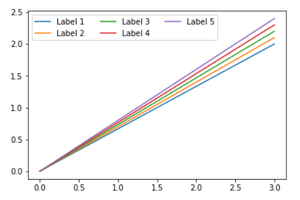GeeksforGeeks App
Open AppBrowser
Continue

# Use multiple columns in a Matplotlib legend

In this article, the task is to use multiple columns in a Matplotlib legend in Python. Before starting the discussion on “Use multiple columns in a Matplotlib legend”, firstly we should know briefly about matplotlib, pyplot and legend.

• Matplotlib: The “matplotlib” library basically used to create so many graphical things such like high quality graphs, charts, figures and many things. The library is extensive and capable of changing very small to the smallest details of a figure. This library was introduced by John Hunter and his team in 2002.
• Pyplot:  In Python, “pyplot” is a plotting library used for 2-dimensional graphics. It is used in python scripts, shell, web application servers and other GUI toolkits.
• Legend: A legend is an area that describes the elements of a graph. In the matplotlib, there is a function called legend() which is used to place a legend on the mentioned axis.

Note: Before declaring matplotlib and pyplot, it is better to declare numpy library also.

Basically, we can import pyplot with matplotlib as we generally import other libraries in python, such like

`import matplotlib.pyplot as plt`

or

`from matplotlib import pyplot as plt`

## Use multiple column in a Matplotlib Legend

In many cases, the main thing we may have to encounter that as the legend number gets increased, it may consume a lot of vertical spaces which may create problem for visualization of the graph.  So, in this situation, we need to organize legend labels into multiple columns. For this, it will be easy to place non-disruptively all the legends.

Using the ncol argument inside plt.legend() in below defined manner to specify the number of columns which the legend should have.

`plt.legend(ncol=k)`

Here, k is the number of columns the legend should have in the graph.

Example:

## Python3

 `# code``import` `matplotlib.pyplot as plt` `plt.plot([``0``, ``3``], [``0``, ``2.0``], label``=``'Label 1'``)``plt.plot([``0``, ``3``], [``0``, ``2.1``], label``=``'Label 2'``)``plt.plot([``0``, ``3``], [``0``, ``2.2``], label``=``'Label 3'``)``plt.plot([``0``, ``3``], [``0``, ``2.3``], label``=``'Label 4'``)``plt.plot([``0``, ``3``], [``0``, ``2.4``], label``=``'Label 5'``)` `# Change the number of columns here``plt.legend(ncol``=``3``)` `plt.show()`

Output:OUTPUT

My Personal Notes arrow_drop_up